# Drawing Survival Curves using 'ggplot2'

Contains the function 'ggsurvplot()' for drawing easily beautiful and 'ready-to-publish' survival curves with the 'number at risk' table and 'censoring count plot'. Other functions are also available to plot adjusted curves for `Cox` model and to visually examine 'Cox' model assumptions.

The survminer R package provides functions for facilitating survival analysis and visualization.

The main functions, in the package, are organized in different categories as follow.

Survival Curves

• ggsurvplot(): Draws survival curves with the 'number at risk' table, the cumulative number of events table and the cumulative number of censored subjects table.

• arrange_ggsurvplots(): Arranges multiple ggsurvplots on the same page.

• ggsurvevents(): Plots the distribution of event's times.

• surv_summary(): Summary of a survival curve. Compared to the default summary() function, surv_summary() creates a data frame containing a nice summary from survfit results.

• surv_cutpoint(): Determines the optimal cutpoint for one or multiple continuous variables at once. Provides a value of a cutpoint that correspond to the most significant relation with survival.

• pairwise_survdiff(): Multiple comparisons of survival curves. Calculate pairwise comparisons between group levels with corrections for multiple testing.

Diagnostics of Cox Model

• ggcoxzph(): Graphical test of proportional hazards. Displays a graph of the scaled Schoenfeld residuals, along with a smooth curve using ggplot2. Wrapper around plot.cox.zph().

• ggcoxdiagnostics(): Displays diagnostics graphs presenting goodness of Cox Proportional Hazards Model fit.

• ggcoxfunctional(): Displays graphs of continuous explanatory variable against martingale residuals of null cox proportional hazards model. It helps to properly choose the functional form of continuous variable in cox model.

Summary of Cox Model

• ggforest(): Draws forest plot for CoxPH model.

Competing Risks

• ggcompetingrisks(): Plots cumulative incidence curves for competing risks.

Install from CRAN as follow:

## ggsurvplot: Drawing survival curves

### Basic plots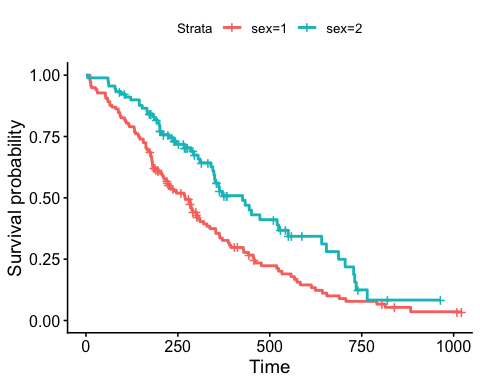Censor shape can be changed as follow:

### Customized survival curves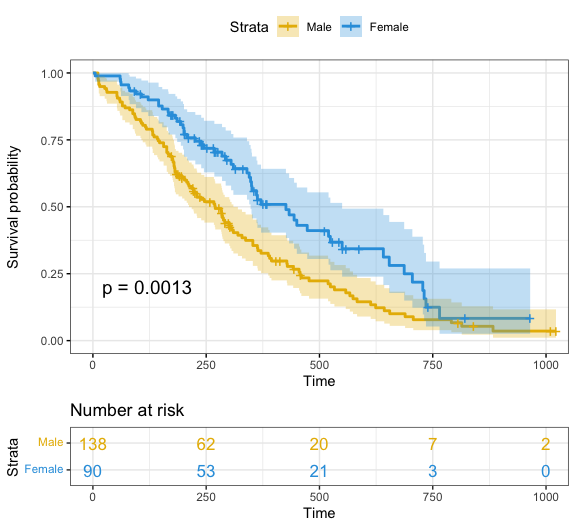Note that, additional arguments are available to customize the main title, axis labels, the font style, axis limits, legends and the number at risk table.

### More customized survival curves

Focus on `xlim` and `break.time.by` parameters which do not change the calculations of estimates of survival surves. Also note `risk.table.y.text.col = TRUE` and `risk.table.y.text = FALSE` that provide bars instead of names in text annotations of the legend of risk table.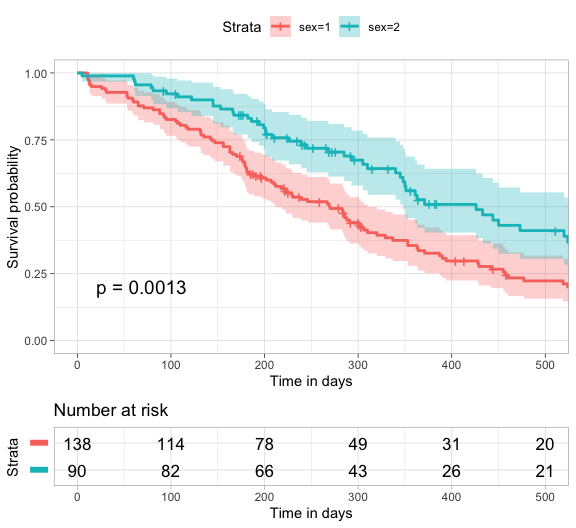### Uber customized survival curves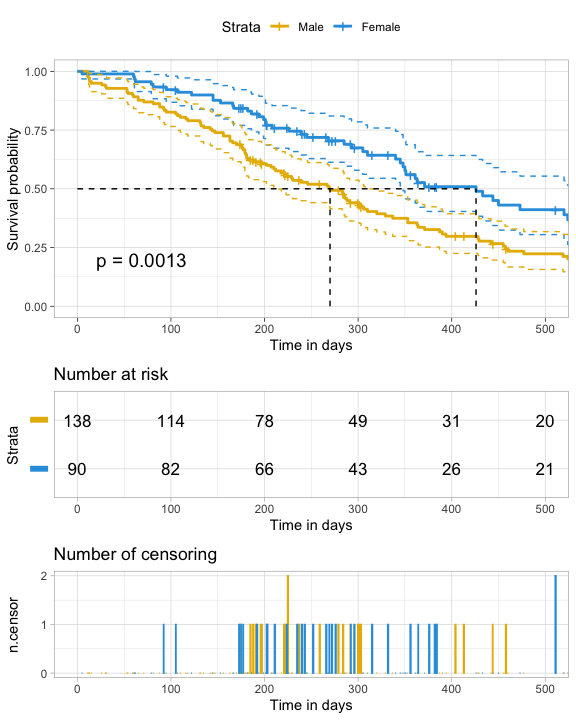### Uber platinum customized survival curves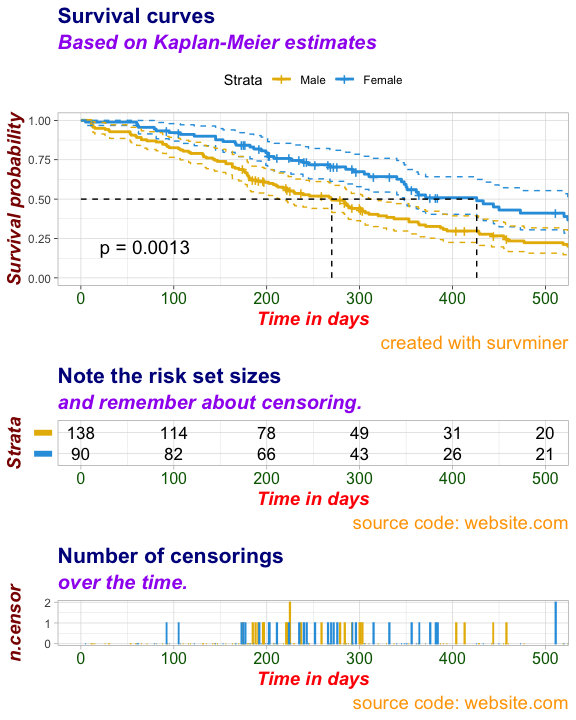## Uber platinum premium customized survival curves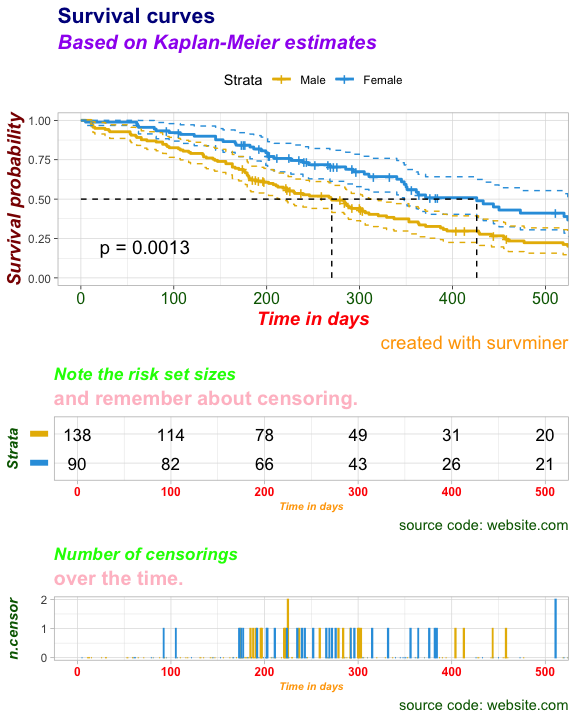# Survminer 0.4.3

## Minor changes

• Now, `ggforest()` simply returns a ggplot instead of drawing automatically the plot (@grvsinghal, #267).

## Bug fixes

• Now, hiding strata names in risk table work when combining survfits (@krassowski, #317).
• Now, `axes.offset` argument is also applied to risk table (@dmartinffm, #243).
• It is now possible to add `ggsurvplot` to powerpoint document using ReporteRs even if there is no risk table (@DrRZ, #314).

# Survminer 0.4.2

## Minor changes

• New argument `size` added in `ggadjustedcurves()` to change the curve size (@MaximilianTscharre, #267).

## Bug fixes

• Now, confidence interval ribbon works properly (@wp07, #275).
• Now, the argument `ggtheme` is supported when combining a list of survfit objects in `ggsurvplot()` (@PhonePong, #278).

# survminer 0.4.1

## New features

• New function `ggflexsurvplot()` to create ggplot2-based graphs for flexible survival models.

• The function `ggadjustedcurves()` handles now argument `method` that defines how adjusted curves shall be calculated. With `method='conditional'|'marginal'` subpopulations are balanced with respect to variables present in the model formula. With `method='single'|'average'` the curve represents just the expected survival curves.

## Major changes

• The function `ggcoxadjustedcurves()` is replaced by `ggadjustedcurves()` (#229).

## Minor changes

• The grouping variable to the `ggadjustedcurves()` function is now passed as a name (character) of grouping variable not as a vector with values of grouping variable.

• New argument `font.family` in `ggsurvtable()` to change the font family in the survival tables - such as risk, cummulative events and censoring tables. For example font.family = "Courier New" (@Swechhya, #245).

• Now, in `ggsurvplot()` the data argument should be strictly provided (@dnzmarcio, #235)

## Bug fixes

• `ggforest()` no longer tries to bolt a table full of text to the coefficient plot (@mmoisse, #241), instead the annotations are done via ggplot2::annotate, see example at: @fabian-s, #264

# survminer 0.4.0

## New features

### New options in ggsurvplot()

• New argument `test.for.trend` added in `ggsurvplot()` to perform a log-rank test for trend. logical value. Default is FALSE. If TRUE, returns the test for trend p-values. Tests for trend are designed to detect ordered differences in survival curves. That is, for at least one group. The test for trend can be only performed when the number of groups is > 2 (#188).

• New argument `add.all` added now in `ggsurvplot()` to add he survival curves of (all) pooled patients onto the main survival plot stratified by grouping variables. Alias of the `ggsurvplot_add_all()` function (#194).

• New argument `combine = TRUE` is now available in the `ggsurvplot()` function to combine a list of survfit objects on the same plot. Alias of the ggsurvplot_combine() function (#195).

• The standard convention of ggplot2 is to have the axes offset from the origin. This can be annoying with Kaplan-Meier plots. New argument `axes.offset` added non in `ggsurvplot()`. logical value. Default is TRUE. If FALSE, set the plot axes to start at the origin (c(0,0)) (#196).

• The function `ggsurvplot()` can take a list of survfit objects and produces a list of ggsurvplots (#204).

• New argument `facet.by` added now in `ggsurvplot()` to draw multi-panel survival curves of a data set grouped by one or two variables. Alias of the `ggsurvplot_facet()` function (#205).

• New argument `group.by` added now in `ggsurvplot()` to create survival curves of grouped data sets. Alias of the `ggsurvplot_group_by()` function.

• In `ggsurvplot()`, one can specify pval = TRUE/FALSE as a logical value. Now, it's also possible to specify the argument `pval` as a numeric value (e.g.: pval = 0.002), that will be passed to the plot, so that user can pass any custom p-value to the final plot (@MarcinKosinski, #189) or one can specify it as a character string (e.g.: pval = "p < 0001") (@MarcinKosinski, #193).

• New argument `xscale` in `ggsurvplot()`: numeric or character value specifying x-axis scale.

• If numeric, the value is used to divide the labels on the x axis. For example, a value of 365.25 will give labels in years instead of the original days.
• If character, allowed options include one of c("d_m", "d_y", "m_d", "m_y", "y_d", "y_m"), where d = days, m = months and y = years. For example, xscale = "d_m" will transform labels from days to months; xscale = "m_y", will transform labels from months to years (#166).
• New arguments `censor.shape` and `censor.size` to change the shape and the shape of censors (#186 & #187).

• New argument `conf.int.alpha` added in `ggsurvplot()`. Numeric value specifying fill color transparency. Value should be in [0, 1], where 0 is full transparency and 1 is no transparency.

### New functions

• New function `surv_group_by()` added to create a grouped data set for survival analysis.

• New function `ggsurvplot_df()` added. An extension to ggsurvplot() to plot survival curves from any data frame containing the summary of survival curves as returned the surv_summary() function. Might be useful for a user who wants to use ggsurvplot for visualizing survival curves computed by another method than the standard survfit.formula function. In this case, the user has just to provide the data frame containing the summary of the survival analysis.

• New function `surv_median()` added to easily extract median survivals from one or a list of survfit objects (#207).

• New function `surv_pvalue`() added to compute p-value from survfit objects or parse it when provided by the user. Survival curves are compared using the log-rank test (default). Other methods can be specified using the argument method.

• New function `surv_fit`() added to handle complex situation when computing survival curves (Read more in the doc: ?surv_fit). Wrapper arround the standard `survfit`() [survival] function to create survival curves. Compared to the standard survfit() function, it supports also:

• a list of data sets and/or a list of formulas,
• a grouped data sets as generated by the function surv_group_by,
• group.by option

## Major changes

• The `ggforest()` function has changed a lot. Now presents much more statistics for each level of each variable (extracted with `broom::tidy`) and also some statistics for the `coxph` model, like AIC, p.value, concordance (extracted with `broom::glance`) (#178)

## Minor changes

• Now, `ggcompetingrisks()` supports the `conf.int` argument. If `conf.int=TRUE` and `fit` is an object of class `cuminc` then confidence intervals are plotted with `geom_ribbon`.

• Now, `ggsurvplot()` supports the `survfit()` outputs when used with the argument `start.time`.

• Now, the default behaviour of `ggsurvplot()` is to round the number at risk using the option `digits = 0` (214).

• `pairwise_survdiff()` has been improved to handle a formula with multiple variables (213).

• The argument `color` are updated allowing to assign the same color for same groups accross facets (#99 & #185).

• If the number of strata/group (n.strata) = 1, the expected value is the color name. For example color = "blue".
• If n.strata > 1, the expected value is the grouping variable name. By default, survival curves are colored by strata using the argument color = "strata", but you can also color survival curves by any other grouping variables used to fit the survival curves.

For example, in the following script, survival curves are colored by the grouping variable `sex` in all facets:

• Now, the function `pairwise_survdiff()` checks whether the grouping variable is a factor. If this is not the case, the grouping variable is automatically converted into a factor.

• `ggsurvplot()`: Now, log scale is used for x-axis when plotting the complementary log−log function (argument `fun = "cloglog") (#171).

• Now, the argument `palette` in `ggsurvplot()` ccan be also a numeric vector of length(strata); in this case a basic color palette is created using the function `grDevices::palette()`.

• The `%+%` function in `survminer` has been replaced by `%++%` to avoid breaking the `ggplot2::%+%` function behavior when using survminer (#199 and #200).

• New argument `fun` added in `ggcoxadjustedcurves()` (@meganli, #202).

• The function `theme_classic2()` removed.

## Bug fixes

• Columns/Rows are now correctly labeled in `pairwise_survdiff`() display (@mriffle, #212).

• Now, the `pairwise_survdiff()` function works when the data contain NAs (@emilelatour , #184).

• Now, `ggsurvplot()` fully supports different methods, in the survMisc package, for comparing survival curves (#191).

# survminer 0.3.1

## Minor changes

• The example section of the `ggcoxdiagnostics()` function and the vignette file `Informative_Survival_Plots.Rmd` have been updated so that `survminer` can pass CRAN check under R-oldrelease.
• New example dataset `BMT` added for competing risk analysis.
• New data set `BRCAOV.survInfo` added, used in vignette files

## Bug fixes

• Now, `palette` argument works in `ggcoxadjustedcurves() (#174)
• Now `ggsurvplot()` works when the `fun` argument is an arbitrary function (#176).

# survminer 0.3.0

## New features

### New options in ggsurvplot()

• Additional `data` argument added to the `ggsurvplot()` function (@kassambara, #142). Now, it's recommended to pass to the function, the data used to fit survival curves. This will avoid the error generated when trying to use the `ggsurvplot()` function inside another functions (@zzawadz, #125).

• New argument `risk.table.pos`, for placing risk table inside survival curves (#69). Allowed options are one of c("out", "in") indicating 'outside' or 'inside' the main plot, respectively. Default value is "out".

• New arguments `tables.height, tables.y.text, tables.theme, tables.col`: for customizing tables under the main survival plot: (#156).

• New arguments `cumevents` and `cumcensor`: logical value for displaying the cumulative number of events table (#117) and the cumulative number of censored subject (#155), respectively.

• Now, `ggsurvplot()` can display both the number at risk and the cumulative number of censored in the same table using the option `risk.table = 'nrisk_cumcenor'` (#96). It's also possible to display the number at risk and the cumulative number of events using the option `risk.table = 'nrisk_cumevents'`.

• New arguments `pval.method` and `log.rank.weights`: New possibilities to compare survival curves. Functionality based on `survMisc::comp`.

• New arguments `break.x.by` and `break.y.by`, numeric value controlling x and y axis breaks, respectively.

• Now, `ggsurvplot()` returns an object of class ggsurvplot which is list containing the following components (#158):

• plot: the survival plot (ggplot object)
• table: the number of subjects at risk table per time (ggplot object). Returned only when risk.table = TRUE.
• cumevents: the cumulative number of events table (ggplot object). Returned only when cumevents = TRUE.
• ncensor.plot: the number of censoring (ggplot object). Returned only when ncensor.plot = TRUE or cumcensor = TRUE.
• data.survplot: the data used to plot the survival curves (data.frame).
• data.survtable: the data used to plot the tables under the main survival curves (data.frame).

### Themes

• New function `theme_survminer()` to change easily the graphical parameters of plots generated with survminer (#151). A theme similar to theme_classic() with large font size. Used as default theme in survminer functions.

• New function `theme_cleantable()` to draw a clean risk table and cumulative number of events table. Remove axis lines, x axis ticks and title (#117 & #156).

### New functions

• New function `+.ggsurv()` to add ggplot components - `theme()`, `labs()` - to an object of class ggsurv, which is a list of ggplots. (#151). For example:
• New function `arrange_ggsurvplots()` to arrange multiple ggsurvplots on the same page (#66).

• New function `ggsurvevents()` to calculate and plot the distribution for events (both status = 0 and status = 1); with `type` parameter one can plot cumulative distribution of locally smooth density; with normalised, distributions are normalised. This function helps to notice when censorings are more common (@pbiecek, #116).

• New function `ggcoxadjustedcurves()` to plot adjusted survival curves for Cox proportional hazards model (@pbiecek, #133 & @markdanese, #67).

• New function `ggforest()` for drawing forest plot for the Cox model.

• New function `pairwise_survdiff()` for multiple comparisons of survival Curves (#97).

• New function `ggcompetingrisks()` to plot the cumulative incidence curves for competing risks (@pbiecek, #168.

### Helper functions

New heper functions `ggrisktable()`, `ggcumevents()`, `ggcumcensor()`. Normally, users don't need to use these function directly. Internally used by the function `ggsurvplot()`.

• `ggrisktable()` for plotting number of subjects at risk by time. (#154).
• `ggcumevents()` for plotting the cumulative number of events table (#117).
• `ggcumcensor()` for plotting the cumulative number of censored subjects table (#155).

## Major changes

• New argument `sline` in the `ggcoxdiagnostics()` function for adding loess smoothed trend on the residual plots. This will make it easier to spot some problems with residuals (like quadratic relation). (@pbiecek, #119).

• The design of `ggcoxfunctional()` has been changed to be consistent with the other functions in the survminer package. Now, `ggcoxfunctional()` works with coxph objects not formulas. The arguments formula is now deprecated (@pbiecek, #115).

• In the `ggcoxdiagnostics()` function, it's now possible to plot Time in the OX axis (@pbiecek, #124). This is convenient for some residuals like Schoenfeld. The `linear.predictions` parameter has been replaced with `ox.scale = c("linear.predictions", "time", "observation.id")`.

## Minor changes

• New argument `tables.height` in `ggsurvplot()` to apply the same height to all the tables under the main survival plots (#157).

• It is possible to specify `title` and `caption` for `ggcoxfunctional` (@MarcinKosinski, #138) (`font.main` was removed as it was unused.)

• It is possible to specify `title`, `subtitle` and `caption` for `ggcoxdiagnostics` (@MarcinKosinski, #139) and `fonts` for them.

• It is possible to specify global `caption` for `ggcoxzph` (@MarcinKosinski, #140).

• In `ggsurvplot()`, more information, about color palettes, have been added in the details section of the documentation (#100).

• The R package `maxstat` doesn't support very well an object of class `tbl_df`. To fix this issue, now, in the `surv_cutpoint()` function, the input data is systematically transformed into a standard data.frame format (@MarcinKosinski, #104).

• It's now possible to print the output of the survminer packages in a powerpoint created with the ReporteRs package. You should use the argument newpage = FALSE in the `print()` function when printing the output in the powerpoint. Thanks to (@abossenbroek, #110) and (@zzawadz, #111). For instance:

• Now, in `ggcoxdiagnostics()`, the option `ncol = 1` is removed from the function `facet_wrap()`. By default, `ncol = NULL`. In this case, the number of columns and rows in the plot panels is defined automatically based on the number of covariates included in the cox model.

## Bug fixes

• Now, risk table align with survival plots when legend = "right" (@jonlehrer, #102).

• Now, `ggcoxzph()` works for univariate Cox analysis (#103).

• Now, `ggcoxdiagnostics()` works properly for schoenfeld residuals (@pbiecek, #119).

• Now, `ggsurvplot()` works properly in the situation where `strata()` is included in the cox formula (#109).

## Vignettes and examples

• A new vignette and a `ggsurvplot` example was added to present new functionalities of possible texts and fonts customizations.

• A new vignette and a `ggsurvplot` example was added to present new functionalities of possible weights specification in a Log-rank test.

# survminer 0.2.4

## Bug fixes

• `surv_summary()` (v0.2.3) generated an error when the name of the variable used in `survfit()` can be found multiple times in the levels of the same variable. For example, variable = therapy; levels(therapy) --> "therapy" and "hormone therapy" (#86). This has been now fixed.

• To extract variable names used in `survival::survfit()`, the R code `strsplit(strata, "=|,\\s+", perl=TRUE)` was used in the `surv_summary()` function [survminer v0.2.3]. The splitting was done at any "=" symbol in the string, causing an error when special characters (=, <=, >=) are used for the levels of a categorical variable (#91). This has been now fixed.

• Now, `ggsurvplot()` draws correctly the risk.table (#93).

# survminer 0.2.3

## New features

• New function `surv_summary()` for creating data frame containing a nice summary of a survival curve (#64).
• It's possible now to facet the output of `ggsurvplot()` by one or more factors (#64):
``````# Fit complexe survival curves
require("survival")
fit3 <- survfit( Surv(time, status) ~ sex + rx + adhere,
data = colon )

# Visualize by faceting
# Plots are survival curves by sex faceted by rx and adhere factors.
require("survminer")
ggsurv\$plot +theme_bw() + facet_grid(rx ~ adhere)
``````
• Now, `ggsurvplot()` can be used to plot cox model (#67).
• New 'myeloma' data sets added.
• New functions added for determining and visualizing the optimal cutpoint of continuous variables for survival analyses:
• `surv_cutpoint()`: Determine the optimal cutpoint for each variable using 'maxstat'. Methods defined for surv_cutpoint object are summary(), print() and plot().
• `surv_categorize()`: Divide each variable values based on the cutpoint returned by `surv_cutpoint()` (#41).
• New argument 'ncensor.plot' added to `ggsurvplot()`. A logical value. If TRUE, the number of censored subjects at time t is plotted. Default is FALSE (#18).

## Minor changes

• New argument 'conf.int.style' added in `ggsurvplot()` for changing the style of confidence interval bands.
• Now, `ggsurvplot()` plots a stepped confidence interval when conf.int = TRUE (#65).
• `ggsurvplot()` updated for compatibility with the future version of ggplot2 (v2.2.0) (#68)
• ylab is now automatically adapted according to the value of the argument `fun`. For example, if fun = "event", then ylab will be "Cumulative event".
• In `ggsurvplot()`, linetypes can now be adjusted by variables used to fit survival curves (#46)
• In `ggsurvplot()`, the argument risk.table can be either a logical value (TRUE|FALSE) or a string ("absolute", "percentage"). If risk.table = "absolute", `ggsurvplot()` displays the absolute number of subjects at risk. If risk.table = "percentage", the percentage at risk is displayed. Use "abs_pct" to show both the absolute number and the percentage of subjects at risk. (#70).
• New argument surv.median.line in `ggsurvplot()`: character vector for drawing a horizontal/vertical line at median (50%) survival. Allowed values include one of c("none", "hv", "h", "v"). v: vertical, h:horizontal (#61).
• Now, default theme of ggcoxdiagnostics() is ggplot2::theme_bw().

## Bug fixes

• `ggcoxdiagnostics()` can now handle a multivariate Cox model (#62)
• `ggcoxfunctional()` now displays graphs of continuous variable against martingale residuals of null cox proportional hazards model (#63).
• When subset is specified in the survfit() model, it's now considered in `ggsurvplot()` to report the right p-value on the subset of the data and not on the whole data sets (@jseoane, #71).
• `ggcoxzph()` can now produce plots only for specified subset of varibles (@MarcinKosinski, #75)

# survminer 0.2.2

## New features

• New `ggcoxdiagnostics` function that plots diagnostic graphs for Cox Proportional Hazards model (@MarcinKosinski, #16).
• Vignette added: `Survival plots have never been so informative` (@MarcinKosinski, #39)
• New argument linetype in 'ggsurvplot' (@MarcinKosinski, #45). Allowed values includes i) "strata" for changing linetypes by strata (i.e. groups); ii) a numeric vector (e.g., c(1, 2)) or a character vector c("solid", "dashed").

# survminer 0.2.1

## New features

• New `ggcoxzph` function that displays a graph of the scaled Schoenfeld residuals, along with a smooth curve using 'ggplot2'. Wrapper around \link{plot.cox.zph}. (@MarcinKosinski, #13)

• New `ggcoxfunctional` function that displays graphs of continuous explanatory variable against martingale residuals of null cox proportional hazards model, for each term in of the right side of input formula. This might help to properly choose the functional form of continuous variable in cox model, since fitted lines with `lowess` function should be linear to satisfy cox proportional hazards model assumptions. (@MarcinKosinski, #14)

• New function `theme_classic2`: ggplot2 classic theme with axis line. This function replaces ggplot2::theme_classic, which does no longer display axis lines (since ggplot2 v2.1.0)

## Minor changes

• post-customization of color and fill no longer shows warnings like "Scale for 'fill' is already present. Adding another scale for 'fill', which will replace the existing scale" (@MarcinKosinski, #11).
• now, post-customization of survival curve colors will automatically affect the risk table y axis text colors (@MarcinKosinski, #11).
• Default value for the argument `risk.table.y.text.col` is now TRUE.
• New argument risk.table.y.text for the function `ggsurvplot`. logical argument. Default is TRUE. If FALSE, risk table y axis tick labels will be hidden (@MarcinKosinski, #28).

# survminer 0.2.0

## New features

• New arguments in ggsurvplot for changing font style, size and color of main title, axis labels, axis tick labels and legend labels: font.main, font.x, font.y, font.tickslab, font.legend.

• New arguments risk.table.title, risk.table.fontsize in ggsurvplot

• New argument risk.table.y.text.col: logical value. Default value is FALSE. If TRUE, risk table tick labels will be colored by strata (@MarcinKosinski, #8).

• `print.ggsurvplot()` function added: S3 method for class 'ggsurvplot'.

• ggsurvplot returns an object of class ggsurvplot which is list containing two ggplot objects:

• plot: the survival plot
• table: the number at risk table per time
• It's now possible to customize the output survival plot and the risk table returned by ggsurvplot, and to print again the final plot. (@MarcinKosinski, #2):

``````# Fit survival curves
require("survival")
fit<- survfit(Surv(time, status) ~ sex, data = lung)

# visualize
require(survminer)
ggsurvplot(fit, pval = TRUE, conf.int = TRUE,
risk.table = TRUE)

# Customize the output and then print
res <- ggsurvplot(fit, pval = TRUE, conf.int = TRUE,
risk.table = TRUE)
res\$table <- res\$table + theme(axis.line = element_blank())
res\$plot <- res\$plot + labs(title = "Survival Curves")
print(res)
``````

## Minor changes

• p < 0.0001 is used (when pvalue < 0.0001).

## Bug fixes

• ggtheme now affects risk.table (@MarcinKosinski, #1)

• xlim changed to cartesian coordinates mode (@MarcinKosinski, #4). The Cartesian coordinate system is the most common type of coordinate system. It will zoom the plot (like you’re looking at it with a magnifying glass), without clipping the data.

• Risk table and survival curves have now the same color and the same order

• Plot width is no longer too small when legend position = "left" (@MarcinKosinski, #7).

# survminer 0.1.1

## New features

• ggsurvplot(): Drawing survival curves using ggplot2

# Reference manual

install.packages("survminer")

0.4.6 by Alboukadel Kassambara, 5 months ago

http://www.sthda.com/english/rpkgs/survminer/

Report a bug at https://github.com/kassambara/survminer/issues

Browse source code at https://github.com/cran/survminer

Authors: Alboukadel Kassambara [aut, cre] , Marcin Kosinski [aut] , Przemyslaw Biecek [aut] , Scheipl Fabian [ctb]

Documentation:   PDF Manual

Depends on ggplot2, ggpubr

Suggests knitr, flexsurv, cmprsk

Imported by MetabolicSurv, concurve, finalfit, ggquickeda, glmaag, survivalAnalysis, survxai.

Depended on by LogrankPower.

Suggested by QTOCen, factorMerger, survParamSim.

See at CRAN